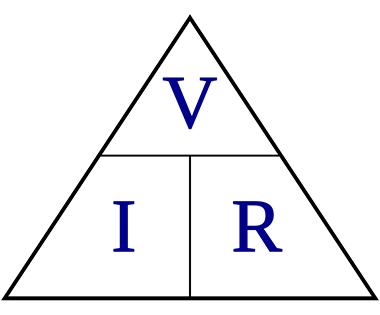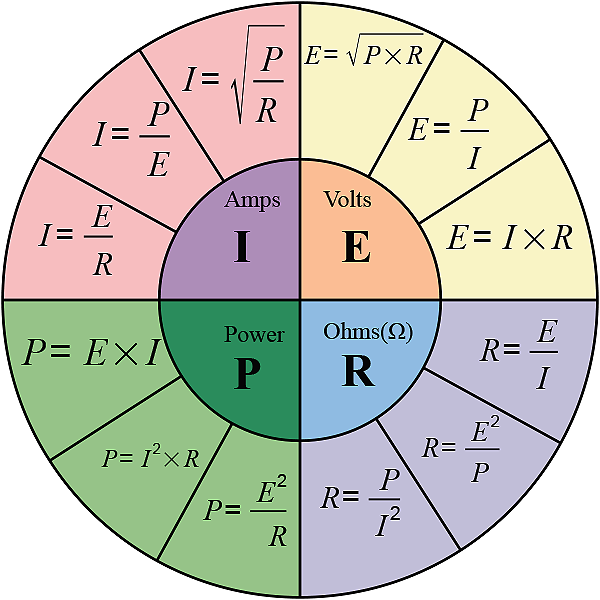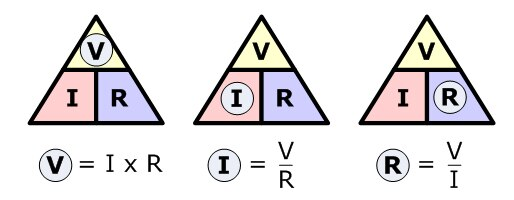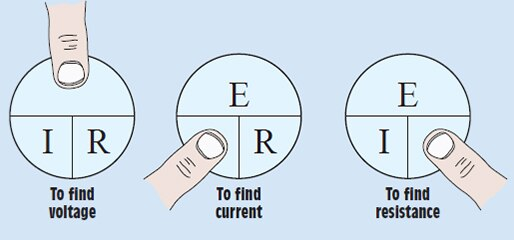### Calculating Watts, Amps and Volts

When beginning to explore the world of electricity and electronics, it is vital to start by understanding the basics of voltage, current, and resistance. These are the three basic building blocks required to manipulate and utilize electricity. The electric potential difference between two points on a circuit is equivalent to the product of the current between those two points and the total resistance of all electrical devices present between those two points, is often referred to as the Ohm's law equation.

## Ohm's Law

Ohm's Law is the mathematical relationship among electric current, resistance, and voltage. The principle is named after the German scientist Georg Simon Ohm. Ohm's Law states that in an electric circuit; the current (I) passing through a conductor varies directly as the potential difference (V) applied at its ends and inversely as the resistance (R) of the conductor; this may be applied to the entire circuit or to a particular part of a circuit.In direct-current (DC) circuits, Ohm's Law is simple and linear. Suppose a resistance having a value of (R) ohms carries a current of (I) amperes. Then the voltage across the resistor is equal to the product (IR). There are two corollaries. If a DC power source providing (E) volts is placed across a resistance of (R) ohms, then the current through the resistance is equal to (E/R) amperes. Also, in a DC circuit, if (E) volts appear across a component that carries (I) amperes, then the resistance of that component is equal to (E/I) ohms.We use the priciples of Ohm's Law to calculate Watts, Amps and Volts:

Watts = Volts x Amps
Amps = Watts / Volts
Volts = Watts / Amps

We may also use Coil Resistance to determine Current:   Amps = Volts / Coil ResistanceIf the math or the concepts are difficult to grasp, you can always find a web page that will do the heavy lifting for you. Here's a link to a helpful Ohm's Law calculator:

http://www.ohmslawcalculator.com/ohms-law-calculator